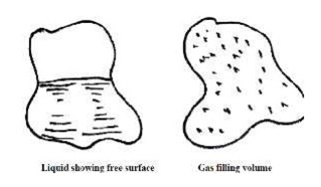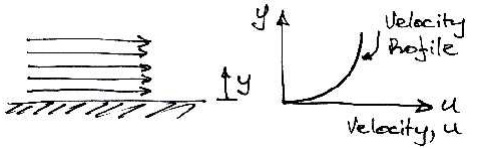Home | | Mechanics of Fluids | Introduction To Fluids

# Introduction To FluidsThere are three states of matter: solids, liquids and gases. Both liquids and gases are classified as fluids.

INTRODUCTION TO FLUIDS

Definition

There are three states of matter: solids, liquids and gases. Both liquids and gases are classified as fluids.

Fluids do not resist a change in shape. Therefore fluids assume the shape of the container they occupy.

Liquids may be considered to have a fixed volume and therefore can have a free surface.

Liquids are almost incompressible.

C o n v e r s e l y , gases are easily compressed and will expand to fill a container they occupy.

We will usually be interested in liquids, either at rest or in motion.Definition

The strict definition of a fluid is: A fluid is a substance which conforms continuously

under the action of shearing forces.

To understand this, remind ourselves of what a shear force is:

2 Static Fluids

According to this definition, if we apply a shear force to a fluid it will deform and take up

a state in which no shear force exists. Therefore, we can say: If a fluid is at rest there can be no shearing forces acting and therefore all forces in the fluid must be perpendicular to the planes in which they act. Note here that we specify that the fluid must be at rest. This is because, it is found experimentally that fluids in motion can have slight resistance to shear force. This is

the source of viscosity.

Fluids in Motion

For example, consider the fluid shown flowing along a fixed surface. At the surface there will be little movement of the fluid (it will 'stick' to the surface), whilst furtheraway from the surface the fluid flows faster (has greater velocity):If one layer of is moving faster than another layer of fluid, there must be shear forcesacting between them. For example, if we have fluid in contact with a conveyor beltthat is moving we will get the behaviour shown:When fluid is in motion, any difference in velocity between adjacent layers has the same effect as the conveyor belt does.

Therefore, to represent real fluids in motion we must consider the action of shear forces

Consider the small element of fluid shown, which is subject to shear force and has a dimension sinto the page. The force F acts over an area A = BC� s. Hence we have a shear stress applied:Study Material, Lecturing Notes, Assignment, Reference, Wiki description explanation, brief detail
Civil - Mechanics Of Fluids - Fluid Properties And Fluid Statics : Introduction To Fluids |

Related Topics